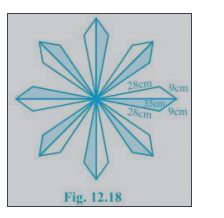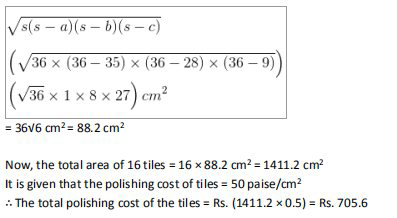# NCERT Solutions Class 9 Mathematics Solutions for Heron’s Formula - Exercise 12.2 in Chapter 12 - Heron’s Formula

A floral design on a floor is made up of 16 tiles which are triangular, the sides of the

the triangle is 9 cm, 28 cm, and 35 cm (see Fig. 12.18). Find the cost of polishing the tiles at the

rate of 50p per \mathrm{cm}^{2}The semi perimeter of the each triangular shape = (28 + 9 + 35)/2 cm = 36 cm

By using Heron's formula,

The area of each triangular shape will beVideo transcript
"hello students welcome to leader q a video session i am seth your math tutor and question for today is a floral design on a floor is made up of 16 tiles which are triangular the sides of the triangle is 9 centimeter 28 centimeter and 35 centimeter you can see the figure 12.18 find the cost of polishing the tiles at the rate of rupees 50 at the rate of 50 passes per centimeter square so here first of all we need to find semi perimeter and then we will try to find area for whole figure so here semi perimeter will be equal to that is 28 plus 9 plus 35 remember this is the semi perimeter of each triangular shape that is 28 plus 9 plus 35 side there three sides are these addition of three sides so divided by two and that is semi parameter s and that is 36 centimeter so s is 36 centimeter by using harold's formula the area of each triangle shape will be root off as s minus a s minus b as minus c that is root of s is 36 36 minus 35 and you have 36 minus 28 and then you have 36 minus 9 so it is root of 36 into 1 into 8 into 27 centimeters square this is our area of each triangle shape so in the next step i'll write down here our answer for this is 36 root 6 centimeter square that will be 88.2 centimeter square now this is area of each triangle shape now the total area of 16 tiles will be equal to 16 into area of one type that is area of each shape let us continue over here 16 into 88.2 that is 1411.2 centimeter square that is the area of 16 times now the cost of per tile polishing is given that is 50 per centimeter square so simply what is the cost of polishing 16 times for that we need to multiply that area of 16 tiles into cost per centimeter square it is 1411.2 into 50 or this 50 is per se so you can write here in rupees as 0.5 rupees and the answer will be coming in rupees now so 1411.2 into 0.5 will be equal to 705.6 rupees hence cost of polishing 16 tiles is equal to 705.6 rupees that is our final answer if you have any query you can post it in our comment section and subscribe to lido for more such q a thank you for watching "
Related Questions

Lido

Courses

Teachers

Book a Demo with us

Syllabus

Maths
CBSE
Maths
ICSE
Science
CBSE

Science
ICSE
English
CBSE
English
ICSE
Coding

Terms & Policies

Selina Question Bank

Maths
Physics
Biology

Allied Question Bank

Chemistry
Connect with us on social media!